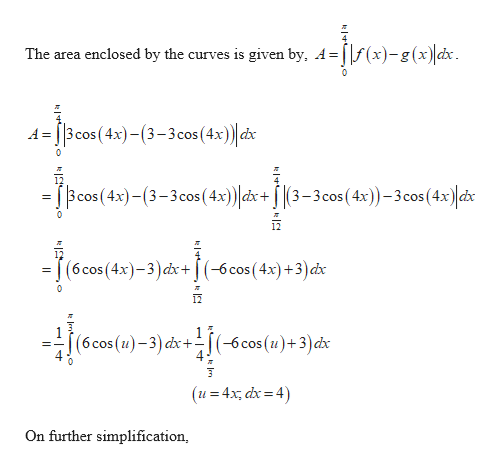# Find the area. y = 3 cos(4x),    y = 3 − 3 cos(4x),    0 ≤ x ≤ π/4

Question
72 views

Find the area.

y = 3 cos(4x),    y = 3 − 3 cos(4x),    0 ≤ x ≤ π/4

check_circle

Step 1
Step 2help_outlineImage TranscriptioncloseThe area enclosed by the curves is given by, A=[f(x)-g(x) dx. A = [3 cos (4x)-(3–3cos(4x)) dx Bcos (4x)-(3-3cos (4x)) dx+ [ |(3– 3 cos ( 4x))– 3cos(4x) dx (6cos(4x)-3)dx+[(-6cos(4x)+3)dx 12 (6cos(u)-3) dx+(-6cos (u)+3)dx (u = 4x, dx = 4) On further simplification, fullscreen

### Want to see the full answer?

See Solution

#### Want to see this answer and more?

Solutions are written by subject experts who are available 24/7. Questions are typically answered within 1 hour.*

See Solution
*Response times may vary by subject and question.
Tagged in

### Calculus